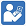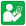Test Sheets - Multiply by 1-Digit Numbers
Multiply by 1-Digit Numbers - Test 1
Multiply by 1-Digit Numbers - Test 2
Multiply by 1-Digit Numbers - Test 3Practice Sheets - Multiply by 1-Digit Numbers